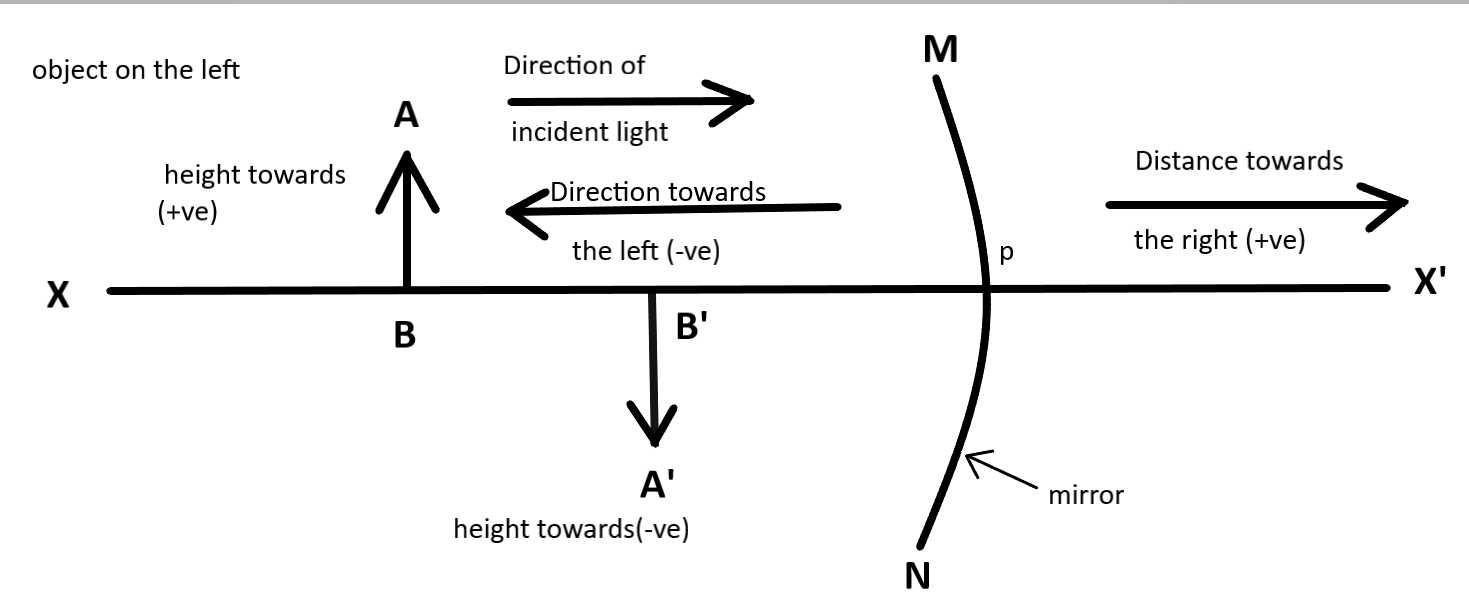Courses
Courses for Kids
Free study material
Offline Centres
MoreLast updated date: 07th Dec 2023
Total views: 281.4k
Views today: 8.81k

# Write the rules for sign convention.Verified
281.4k+ views
Hint: We all know there are two types of mirror – Concave and Convex mirror. To answer this question we have to understand the position of object and image.

Complete step-by-step solution:
Lets see the rule for sign convention:
All distance ought to be measured from the pole.
Distances measured within the direction of incident light to be taken $\left( + \right)ve$ and measured in the backward direction of incident light to be taken $\left( - \right)ve$.
Height of object and height of image measured upward and perpendicular to the principal axis are taken as $\left( + \right)ve$where those downwards measured are $\left( - \right)ve$.
Clockwise angle measure with optic axis are $\left( - \right)ve$and anticlockwise measured as$\left( + \right)ve$
•Note: The focal length and radius of curvature for concave mirror taken as$\left( - \right)ve$ .Whereas , for convex mirror focal distance and radius of curvature are taken as $\left( + \right)ve$.